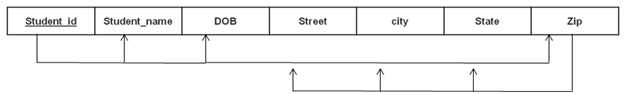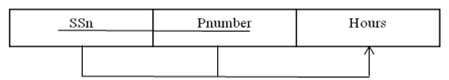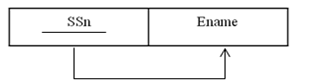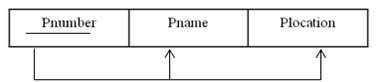Question:
Published on: 23 January, 2022

What is the need for normalization? Explain 2nd and 3rd normal form with example.

Consider the following relation:

EMP_PROJ { SSn, Pnumber, Hours, Ename, Pname, Plocation}

Assume { SSn, Pnumber} as primary key.

The dependencies are {SSn, Pnumber}→ Hours; SSn →Ename; Pnumber → {Pname, Plocation}.

Normalize the above relation into 2NF.

Objectives of Normalization

• Develop a good description of the data, its relationships and constraints
• Produce a stable set of relations that
• Is a faithful model of the enterprise
• Is highly flexible
• Reduces redundancy-saves space and reduces inconsistency in data
• Is free of update, insertion and deletion anomalies

Second normal form (2NF)

A table is said to be in 2NF if both the following conditions hold:

• Table is in 1NF (First normal form)
• No non-prime attribute is dependent on the proper subset of any candidate key of table.

An attribute that is not part of any candidate key is known as non-prime attribute.

Consider the Table1 in First Normal Form, while the candidate key is {Student, Subject}, Age of Student only depends on Student column, Student → Age, which is incorrect as per Second Normal Form. To achieve second normal form, it would be helpful to split out the subjects into an independent table, and match them up using the student names as foreign keys.

Table1

 Student Age Subject Adam 15 Biology Adam 15 Maths Alex 14 Maths Stuart 17 Maths

After splitting, there are two tables,

Table 1:

 Student Age Adam 15 Alex 14 Stuart 17

Table 2

Third Normal Form (3NF)

Third Normal form applies that every non-prime attribute of table must be dependent on primary key, or we can say that, there should not be the case that a non-prime attribute is determined by another non-prime attribute. So this transitive functional dependency should be removed from the table and also the table must be in Second Normal form.In this table Student_id is Primary key, but street, city and state depends upon Zip. The dependency between zip and other fields is called transitive dependency. Hence to apply 3NF, we need to move the street, city and state to new table, with Zip as primary key.

New Student_Detail Table:

 Student_id Student_name DOB Zip

 Zip Street city state

After removing the partial dependencies EMP_PROJ table, there will be three tables,

as below,

• Table 1 :• Table 2 :• Table 3 :Random questions